## 29 Mar 2017

### Kaufmann (1.3) Introduction to the Theory of Fuzzy Subsets, “Le concept de sous-ensemble flou” / “The Concept of a Fuzzy Subset”

[Search Blog Here. Index tabs are found at the bottom of the left column.]

[Central Entry Directory]

[Logic and Semantics, Entry Directory]

[Arnold Kaufman, entry directory]

[Kaufmann’s Introduction to ... Fuzzy Subsets, entry directory]

[The following is summary. Unless otherwise noted, boldface is my own. Page citations refer to the French edition first / then the English. I apologize in advance for my distracting typos or other mistakes, because proofreading is incomplete.]

Summary of

Arnold Kaufmann

Introduction à la théorie des sous-ensembles flous

à l’usage des ingénieurs

(Fuzzy sets theory)

1. Eléments théoriques de base

/

Introduction to the Theory of Fuzzy Subsets.

Vol.1 Fundamental Theoretical Elements

Ch.1

Notions de base

Fundamental Notions

1.3

Le concept de sous-ensemble flou

The Concept of a Fuzzy Subset

Brief summary:

A fuzzy subset is one where the members admit of a variety of degrees of membership, ranging from 0 for not at all a member to 1 for fully a member, with all the decimal values between for the varying degrees of membership. We use a wavy line under the set name to designate it as a fuzzy subset, or we may use the wavy line under the set inclusion symbol.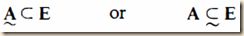We can define the members by assigning to them the set of values, partial or full, from a set M.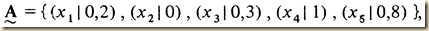Fuzzy membership could also be written using the wavy line under the membership symbol.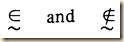We can also designate the degree of membership by writing it under the membership symbol.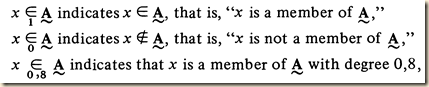Fuzzy subsets allow us to define imprecise concepts, like the fuzzy subset of integers very near 0. As we move away from 0, the membership values will decrease.

Summary

[In the prior section 1.2, Kaufman had an example of two sets:

E = {x1, x2, x3, x4, x5}

A = {x2, x3, x5}

(Here the E seems to operate like a domain of discourse). We can notate this using a valuation function μ which assigns 1 when a member is in a set and 0 when it is not, so:

μA(x) = 1 if xA

= 0 if xA

We can then designate the memberships for A as:

A = μA(x1) = 0,   μA(x2) = 1, μA(x3) = 1,  μA(x4) = 0, μA(x5) = 1

We can also write these as pairs in this way:

A = {(x1, 0), (x2, 1), (x3, 1), (x4, 0), (x5, 1)}

(page 2).] In the example [see above] from the prior section, we designated members of E as either belonging to A or not belonging to A. The valuation function μ (or “characteristic function” as it is called here) only takes one of two values, 0 and 1. (p.4/4)

Kaufmann then has us think that the valuation function μ can take any value between 0 and 1. This allows for partial membership in the subset.

Imagine now that this characteristic function may take any value whatsoever in the interval [0, 1]. Thus, an element xi of E may not be a member of A (μA = 0), could be a member of A a little (μA near 0), may more or less be a member of A (μA neither too near 0 nor too near 1), could be strongly a member of A (μA near 1), or finally might be a member of A (μA = 1). In this manner the notion of membership takes on an interesting extension and leads, as we shall see, to very useful developments.

(4/4)

Such subsets are called fuzzy subsets, and they are notated by either placing a wavy line under the subset name or under the subset symbol. The degree of membership is then notated by writing the value under the membership symbol. [Perhaps we might say that we deal with fuzzy subsets rather than fuzzy sets, because we always need a domain of discourse whose members are established, and then these fully established members in the domain can then be said to be partial members of fuzzy subsets.]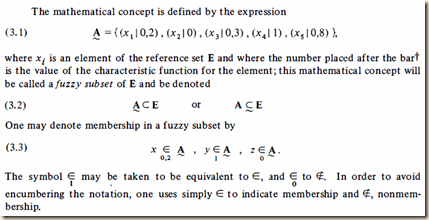(4/4)

So consider the fuzzy subset 3.1 above. We see that some members are only partially so. [His next point seems to be that we can use fuzzy subsets when dealing with vague predicates where there is still a scale of fittingness for that predicate. Let me quote.]

Thus, the fuzzy subset defined by (3.1) contains a little x1, does not contain x2, contains a little more x3, contains x4 completely, and a large part of x5. This will allow us to construct a mathematical structure with which one may be able to manipulate concepts that are rather poorly defined but for which membership in a subset is somewhat hierarchical. Thus, one may consider: in the set of men, the fuzzy subset of very tall men; in the set of basic colors, the fuzzy subset of deep green colors; in the set of decisions, a fuzzy subset of good decisions; and so forth. We shall go on to see how to manipulate these concepts that seem particularly well adapted to the imprecision prevalent in the social sciences.

(4/4)

Kaufmann then gives Zadah’s rigorous definition, Z1. [Let us look first at the example, as it can help understand the structures.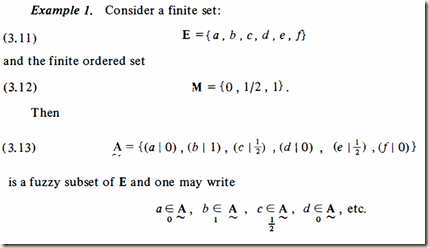(6/6)

Here we see that E is like the domain of discourse, because it has all the possible members. M is the set of membership degrees or values that any member can take. We then define a fuzzy subset A by giving ordered pairs, where the first of the pair is the member name, and the second is the assigned partial value. For some reason, each pairing is enclosed in parentheses and separated by a vertical line. As you will see, there is a special symbol for the function that assigns the partial value to the fuzzy subset member. But I think I am misreading the notation for (3.4). The second member of the pair is ∀x∈E. But I do not know exactly what that would be, as something you could designate as a second member of an ordered pair. It does not seem to show in the examples. Please consult the text below to interpret it for yourself.]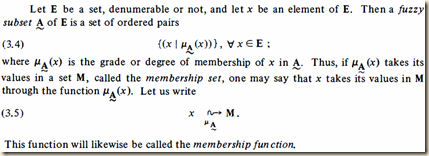(5/5)

[Kaufmann then restructures the above definition for Boolean functions. I am not sure what the important differences are.]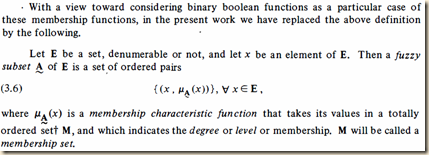(5/5)

Of course: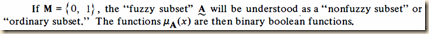(5/5)

Kaufmann then notes that “Thus, the notion of fuzzy subset is linked with the notion of a set and allows one to study, using mathematical structures, imprecise concepts” (5/5).

He next gives some examples for such imprecise concepts:

the fuzzy subset of numbers x approximately equal to a given real number n, where nR (R being the set of reals);

the fuzzy subset of integers very near 0;

let a be a real number and let x be a small positive increment given to a; then the numbers a + x form a fuzzy subset in the set of reals;

let H be an element of a lattice; the elements most near to H in the order relation form a fuzzy subset in the set of elements of the lattice.

(5/5, boldface his)

Kaufmann will use boldface to designate sets or subsets, and the wavy line under them to designate fuzzy subsets.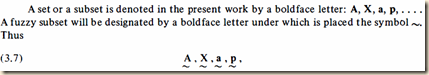(5/5)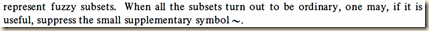(6/6)

Kaufmann then uses these symbols to notate fuzzy membership.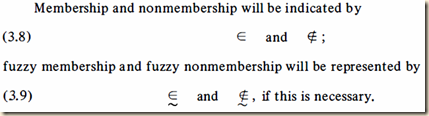(6/6)

We may also write the degree of membership under the membership symbol.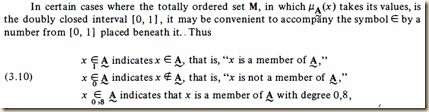(6/6)

[Kaufmann then illustrates with three examples. The first one we already examined above. The second one is interesting, because it might remind us of Nolt’s example of a vague predicate, where each iteration of a statement in which we increase a figure by 1 we also decrease the truth value by a small amount. See Nolt Logics section 16.1.]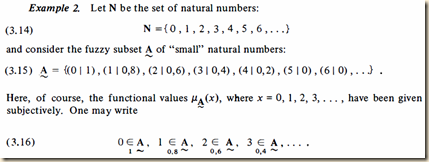(6/6)

[Example three introduces some alternate notation. See p.7/7.]

From:

Kaufmann, Arnold. 1975 . Introduction à la théorie des sous-ensembles flous à l’usage des ingénieurs (Fuzzy sets theory). 1: Eléments théoriques de base. Foreword by L.A. Zadeh. 2nd Edn. Paris: Masson.

Kaufmann, Arnold. 1975. Introduction to the Theory of Fuzzy Subsets. Vol.1: Fundamental Theoretical Elements. Foreword by L.A. Zadeh. English translation by D.L. Swanson. New York / San Francisco / London: Academic Press.

.

### Kaufmann (1.2) Introduction to the Theory of Fuzzy Subsets, “Rappel sur la notion d’appartenance” / “Review of the Notion of Membership”

[Search Blog Here. Index tabs are found at the bottom of the left column.]

[Central Entry Directory]

[Logic and Semantics, Entry Directory]

[Arnold Kaufman, entry directory]

[Kaufmann’s Introduction to ... Fuzzy Subsets, entry directory]

[The following is summary. Unless otherwise noted, boldface is my own. Page citations refer to the French edition first / then the English. I apologize in advance for my distracting typos or other mistakes, because proofreading is incomplete.]

Summary of

Arnold Kaufmann

Introduction à la théorie des sous-ensembles flous

à l’usage des ingénieurs

(Fuzzy sets theory)

1. Eléments théoriques de base

/

Introduction to the Theory of Fuzzy Subsets.

Vol.1 Fundamental Theoretical Elements

Ch.1

Notions de base

Fundamental Notions

1.2

Rappel sur la notion d’appartenance

Review of the Notion of Membership

Brief summary:

Set A being a subset of E is written:

A ⊂ E

x being a member of A is written:

x ∈ A

We can also describe membership using a valuation function μ:

μA(x) = 1 if xA

= 0 if xA

The complement of a set is notated by writing a line above its symbol. We can calculate whether or not some element is a member of a union or intersection of sets by using Boolean operations.

μA ∩B(x) = μA (x) .  μB(x)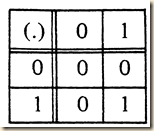μA ∪B(x) = μA (x) + μB(x)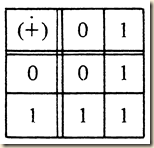Summary

[Kaufmann will define membership and subset inclusion. See especially Suppes’ Introduction to Logic sections 9.1-9.2 and section 9.3 for another great introduction to the concepts and notation.]

We suppose that E is a set and A is a subset of E. We then would write:

A ⊂ E.

(Kaufmann p.1/1). [This symbol is used to mean a proper subset, in Suppes’ text; (if all the members of one set are included in a second, but not all of the second are included in the first, then we call the first a proper subset of the second)]

Suppose E has an element x. If it is a member of A, we write it:

x A.

[The next idea seems to be that we can describe membership using a valuation function.]

In order to indicate this membership one may also use another concept, a characteristic function μA(x), whose value indicates (yes or no) whether x is a member of A:

μA(x) = 1 if xA

= 0 if xA.

(p.1/1)

Kaufmann then gives an example. Here we have two sets with members:

E = {x1, x2, x3, x4, x5}

A = {x2, x3, x5}

(As you can see, the E seems to function like a domain of discourse).

We can then designate the memberships for A as:

A = μA(x1) = 0,   μA(x2) = 1, μA(x3) = 1,  μA(x4) = 0, μA(x5) = 1

We can also write these as pairs in this way:

A = {(x1, 0), (x2, 1), (x3, 1), (x4, 0), (x5, 1)}

(2/2)

Kaufmann next has us consider Boolean binary algebra. [Recall the following from Suppes’ Introduction section 9.5:

Certain operations can be performed on sets. If we find all the members shared in common between two sets, we are finding their intersection ():

(x)(x A B xA & xB)

When two intersecting sets share no members in common, that is, when they are mutually exclusive sets, their intersection is the empty set. The set containing all the members in total from two sets is their union ():

(x)(x ABx A xB)

All the members in set A that are not in set B is the difference () of A and B.

(x)(x A B x A & x B)

And recall the following from section 9.6. A domain of individuals (also called a domain of discourse) is a specific set.  We use the symbol “V” to denote a domain. Suppose we have a domain V and a set A. The complement of A relative to the domain are all those items in the domain that are not in A. We symbolize it either as V∼A or just ∼A. In section 9.9 he noted a number of identities (here Λ is the empty set and ~ is the complement):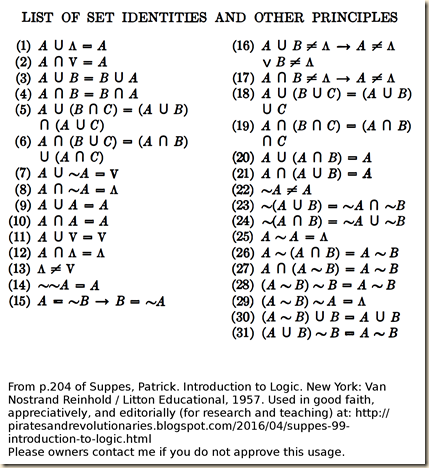] [In the following, I cannot duplicate the notation using typographical symbols, so I will paste images for certain parts. A set’s complement has all the members in the domain that the first set lacks. The intersection of sets are all those members shared by both sets. Thus the intersection of a set and its complement will be empty. The union of sets are all those found in either set. So the union of a set and its complement will be all those in the domain.]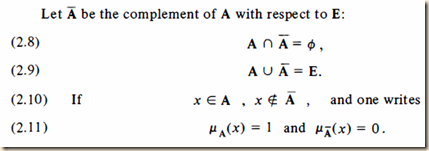(2/2)

Kaufmann next will examine intersections in terms of Boolean products. We first consider two subsets, A and B, and their intersection A ∩ B. We thus can make the following determinations:

μA(x) = 1 if xA

= 0 if xA ,

μB(x) = 1 if xB

= 0 if x ∉ B ,

μA ∩ B(x) = 1 if x ∈ A ∩ B

= 0 if xA ∩ B.

(2/2)

[The next piece of notation is a ‘.’, which seems aligned not above the base-line but right at it, like a period. I am not sure what it means at this point. I first I thought it was like arithmetical multiplication, which would hold in this case, but the ‘+’ operation to follow does not add the 1 values to get 2. It instead seems to be an operator that simply should be understood as conjunction, and + as disjunction.]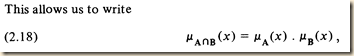(2/2)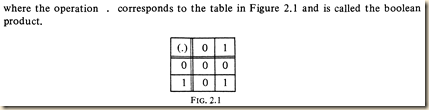(3/3)

We then define the union of two subsets A and B by using the ‘+’ or Boolean sum operator [which seems to correspond to disjunction.]

μA ∪B(x) = 1 if x ∈ A ∪ B

= 0 if x ∉ A ∪ B

μA ∪B(x) = μA (x) + μB(x)

or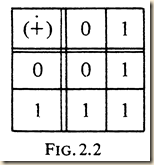(3/3)

[Kaufmann then gives more examples of these operations and also adds to them complementarity. See page 3/3.]

From:

Kaufmann, Arnold. 1975 . Introduction à la théorie des sous-ensembles flous à l’usage des ingénieurs (Fuzzy sets theory). 1: Eléments théoriques de base. Foreword by L.A. Zadeh. 2nd Edn. Paris: Masson.

Kaufmann, Arnold. 1975. Introduction to the Theory of Fuzzy Subsets. Vol.1: Fundamental Theoretical Elements. Foreword by L.A. Zadeh. English translation by D.L. Swanson. New York / San Francisco / London: Academic Press.

.

### Kaufmann (1.1) Introduction to the Theory of Fuzzy Subsets, “Introduction”

[Search Blog Here. Index tabs are found at the bottom of the left column.]

[Central Entry Directory]

[Logic and Semantics, Entry Directory]

[Arnold Kaufman, entry directory]

[Kaufmann’s Introduction to ... Fuzzy Subsets, entry directory]

[The following is summary. Unless otherwise noted, boldface is my own. Page citations refer to the French edition first / then the English. I apologize in advance for my distracting typos or other mistakes, because proofreading is incomplete.]

Summary of

Arnold Kaufmann

Introduction à la théorie des sous-ensembles flous

à l’usage des ingénieurs

(Fuzzy sets theory)

1. Eléments théoriques de base

/

Introduction to the Theory of Fuzzy Subsets.

Vol.1 Fundamental Theoretical Elements

Ch.1

Notions de base

Fundamental Notions

1.1

Introduction

Brief summary:

We will deal with fuzzy subsets and not fuzzy sets, starting first with a review of sets.

Summary

Kaufmann will first review basic notions regarding sets, because we will apply or modify many of these notions when describing fuzzy subsets (1/1).

Kaufmann will proceed slowly for those less adept with mathematics (1/1).

The reader can check their understanding by examining the examples. But Chapter 1 will not be the challenging part. It gets difficult starting with the second chapter (1/1).

As we will see, we will deal with fuzzy subsets and not fuzzy sets. This theory is useful. Although what this theory does can be accomplished with other concepts, it is most effectively expressed in terms of fuzziness (1/1).

From:

Kaufmann, Arnold. 1975 . Introduction à la théorie des sous-ensembles flous à l’usage des ingénieurs (Fuzzy sets theory). 1: Eléments théoriques de base. Foreword by L.A. Zadeh. 2nd Edn. Paris: Masson.

Kaufmann, Arnold. 1975. Introduction to the Theory of Fuzzy Subsets. Vol.1: Fundamental Theoretical Elements. Foreword by L.A. Zadeh. English translation by D.L. Swanson. New York / San Francisco / London: Academic Press.

.

### Kaufmann (symbols list) Introduction to the Theory of Fuzzy Subsets, “Liste des principaux symboles” / “List of Principal Symbols”

[Search Blog Here. Index tabs are found at the bottom of the left column.]

[Central Entry Directory]

[Logic and Semantics, Entry Directory]

[Arnold Kaufman, entry directory]

[Kaufmann’s Introduction to ... Fuzzy Subsets, entry directory]

[The following presents the symbols Kaufmann uses in his book. It is presented here for the sake of commentary on his text (see the other entries here), to be used for research and teaching. As the symbols do not exist typographically, they are reproduced photographically here. Owners please contact me if you do not approve of this usage.]

Introduction à la théorie des sous-ensembles flous

à l’usage des ingénieurs

(Fuzzy sets theory)

1. Eléments théoriques de base

/

Introduction to the Theory of Fuzzy Subsets.

Vol.1 Fundamental Theoretical Elements

Liste des principaux symboles

List of Principal SymbolsKaufmann, Arnold. 1975 . Introduction à la théorie des sous-ensembles flous à l’usage des ingénieurs (Fuzzy sets theory). 1: Eléments théoriques de base. Foreword by L.A. Zadeh. 2nd Edn. Paris: Masson.

Kaufmann, Arnold. 1975. Introduction to the Theory of Fuzzy Subsets. Vol.1: Fundamental Theoretical Elements. Foreword by L.A. Zadeh. English translation by D.L. Swanson. New York / San Francisco / London: Academic Press.

.

### Kaufmann (preface) Introduction to the Theory of Fuzzy Subsets, “Avertissement” / “Preface”

[Search Blog Here. Index tabs are found at the bottom of the left column.]

[Central Entry Directory]

[Logic and Semantics, Entry Directory]

[Arnold Kaufman, entry directory]

[Kaufmann’s Introduction to ... Fuzzy Subsets, entry directory]

[The following is summary. Unless otherwise noted, boldface is my own. Page citations refer to the French edition first / then the English. I apologize in advance for my distracting typos or other mistakes, because proofreading is incomplete.]

Summary of

Arnold Kaufmann

Introduction à la théorie des sous-ensembles flous

à l’usage des ingénieurs

(Fuzzy sets theory)

1. Eléments théoriques de base

/

Introduction to the Theory of Fuzzy Subsets.

Vol.1 Fundamental Theoretical Elements

Avertissement

Preface

(by Arnold Kaufmann)

Brief summary:

The elements of fuzzy subsets are members in an “uncertain fashion” rather than in the certain fashion of classical sets where elements either are or are not in the set. The theory worked out here is about fuzzy subsets and not fuzzy sets, because “the reference set will always be an ordinary set, that is, [...] a collection of well-specified and distinct objects” (xii/xiii-xiv). This theory of fuzzy subsets will prove especially useful for designing intelligent machines so that they can handle fuzzy information, like human minds can.

Summary

Kaufmann notes that our scientific knowledge of the world is limited to the models, representations, “more or less true” laws, and “acceptable approximations in the state of our knowledge” that we use to study the world (ix/xi). [I am not sure about his next point, so I will quote it. It might be that the only confirmation we have of one model is that made by means of another model, and although they continue to correct one another, there will not be a perfect representation of the world, at least until some great revolution in ideas allows for a better means of representation. Here is the quotation:]

And the model of something for one is not exactly the same model of this thing for another; the formula may remain the same, but the interpretation may be different. The universe is perceived with the aid of models that are indeed perfecting themselves through embodying one in another, at least until some revolution in ideas appears, no longer permitting a correct embodyment.

(ix/xi)

But human thinking, unlike computer cognition, is fuzzy. This is partly because we use global or parallel reasoning which is necessarily fuzzy (ix/xi). And there is a lot of room for alterations and adaptation in human learning (ix/xi).

Kaufmann then wonders how we might introduce this real fuzziness into our mathematical systems (ix/xi).

Kaufmann then distinguishes classical and fuzzy membership.

For a mathematician, what does the word fuzzy signify (or synonymous words)? This will mean that an element is a member of a subset only in an uncertain fashion; while, on the other hand, in mathematics we understand that there are only two acceptable situations for an element: being a member of or not being a member of a subset. Any normal logic, boolean logic, rests on this base: membership or nonmembership in a subset of a reference set.

(x/xi, italics his)

L.A. Zadeh’s innovation was to allow for “weighted membership. An element may then belong more or less to a subset, and, from there, introducing the fundamental concept, that of a fuzzy subset” (x/xii).

The multivalued or n-ary logics of Post (1921), Lukasiewicz (1937), and Moisil (1940) opened the way for fuzzy logic. The two schools of fuzzy logic that emerged are of Zadeh and Moisil (x/xii).

One objection to fuzzy logic is that what it accomplishes can be accomplished by other systems. But this objection can be raised for almost any important system. [That in itself does not diminish the value of any system, so it should not diminish the value of fuzzy logic.] (x/xii)

The theory of fuzzy subsets should be of great interest to scientists who study fuzzy systems like language and thought, but also to “the literati and artists, those who construct truth and beauty with fuzziness” (x/xii).

This book is designed to be as accessible as possible for those with a technical interest in the field (x/xii).

Kaufmann added many examples, although for some readers that might make the book too lengthy (xi/xii-xiii).

Fuzziness is here limited to variables and configurations, but one could extrapolate from this presentation other conceptual aspects of fuzziness. So, many disciplines could derive value from this material. (xi/xiii).

Linear computation machines will be able to handle the fuzzy problems we deal with in this book (xi-xii/xiii).

Kaufmann then addresses the question, why do we use the term fuzzy “subset” and not fuzzy “set”? He explains that this is because “the reference set will always be an ordinary set, that is, such as one defined intuitively in modern mathematics, that is again, a collection of well-specified and distinct objects. It is the subsets that will be fuzzy, as we shall see” (xii/xiii-xiv).

Volume 1 presents the theory, while Volume 2 applies it to such areas as “fuzzy languages, fuzzy systems, fuzzy automata, fuzzy algorithms, machines and control, decision problems in a fuzzy universe, recognition of forms, problems of classification and selection, documentary research, etc.” (xii/xiv).

Kaufmann then thanks a number of people who helped with the production of this book (xii/xiv).

He especially thanks his son Alain for his corrections (xii/xiv).

Kaufmann notes that the human mind will “remain fuzzy and creative” (xii/xiv). [The French Avertissement ends here, and then there begins another one for the second edition. Part of that is found in English edition as a continuation of its Preface.]

Kaufmann then notes that he corrected a number of errors for this second edition (xiii/xiv). In the French edition he mentions some features of the text, like the extensive Bibliography, also his new volumes, and he calls upon his readers to work together on furthering our knowledge by means of these findings (xiii).

From:

Kaufmann, Arnold. 1975 . Introduction à la théorie des sous-ensembles flous à l’usage des ingénieurs (Fuzzy sets theory). 1: Eléments théoriques de base. Foreword by L.A. Zadeh. 2nd Edn. Paris: Masson.

Kaufmann, Arnold. 1975. Introduction to the Theory of Fuzzy Subsets. Vol.1: Fundamental Theoretical Elements. Foreword by L.A. Zadeh. English translation by D.L. Swanson. New York / San Francisco / London: Academic Press.

.

## 28 Mar 2017

### Zadeh (Foreword) in Kaufmann Introduction to the Theory of Fuzzy Subsets, “Préface” / “Foreword”

[Search Blog Here. Index tabs are found at the bottom of the left column.]

[Central Entry Directory]

[Logic and Semantics, Entry Directory]

[Arnold Kaufman, entry directory]

[Kaufmann’s Introduction to ... Fuzzy Subsets, entry directory]

[The following is summary. Unless otherwise noted, boldface is my own. Page citations refer to the French edition first / then the English. I apologize in advance for my distracting typos or other mistakes, because proofreading is incomplete.]

Summary of

Arnold Kaufmann

Introduction à la théorie des sous-ensembles flous

à l’usage des ingénieurs

(Fuzzy sets theory)

1. Eléments théoriques de base

/

Introduction to the Theory of Fuzzy Subsets.

Vol.1 Fundamental Theoretical Elements

Foreword

Brief summary:

Fuzzy sets are “classes with unsharp boundaries in which the transition from membership to nonmembership is gradual rather than abrupt” (Zadeh ix). The reliance on classical sets in studies of human life and in human or artificial cognition has limited these efforts, because the real world and human thinking involve fuzziness.

Summary

The theory of fuzzy subsets tries to bring together precise mathematics with the “pervasive imprecision of the real world” (Zadeh v/ix). This is also an effort to better understand mental cognition.

At the time of this writing, artificial intelligence science has been unable to replicate the many types of human cognition (v/ix).

The reason for this is that human cognition has the ability to process imprecise data, while computers do not (v-vi/ix).

“The fundamental concept in mathematics is that of a set – a collection of objects” (vi/ix). However, Zadeh thinks that most human cognition uses fuzzy sets or subsets:

We have been slow in coming to the realization that much perhaps most, of human cognition and interaction with the outside world involves constructs which are not sets in the classical sense, but rather “fuzzy sets” (or subsets), that is, classes with unsharp boundaries in which the transition from membership to nonmembership is gradual rather than abrupt. Indeed, it may be argued that much of the logic of human reasoning is not the classical two-valued or even multivalued logic but a logic with fuzzy truths, fuzzy connectives, and fuzzy rules of inference.

(vi/ix)

Because we have sought precision in our scientific endeavors, we have tried to make the real world fit into mathematical models that leave no room for fuzziness. We have even tried to use such precision to understand human individual and social behavior. Zadeh thinks this is a doomed project (vi/ix).

In our quest for precision, we have attempted to fit the real world to mathematical models that make no provision for fuzziness. We have tried to describe the laws governing the behavior of humans, both singly and in groups, in mathematical terms similar to those employed in the analysis of inanimate systems. This, in my view, has been and will continue to be a misdirected effort, comparable to our long-forgotten searches for the perpetuum mobile and the philosopher’s stone.

(vi/ix)

Instead, Zadeh argues that we need to incorporate fuzziness into our concepts and techniques for studying reality and human life (ix).

What we need is a new point of view, a new body of concepts and techniques in which fuzziness is accepted as an all pervasive reality of human existence. Clearly, we need an understanding of how to deal with fuzzy sets within the framework of classical mathematics. More important, we have to develop novel methods of treating fuzziness in a systematic – but not necessarily quantitative – manner. Such methods could open many new frontiers in psychology, sociology, political science, philosophy, physiology, economics, | linguistics, operations research, management science, and other fields, and provide a basis for the design of systems far superior in artificial intelligence to those we can conceive today.

(vi-vii/ix-x)

Ladeh then praises Kaufmann’s text. It is thorough and lucid, and it is the “first systematic exposition” of fuzzy subset theory (vii/x).

This text will deal with the mathematical aspects of fuzzy subsets, and it should prove useful to engineers and artificial intelligence programmers, because among other things, it details the notion of fuzzy algorithms (vii/x).

Zadeh thinks this book will prove highly influential (x).

From:

L.A. Zadeh’s “Préface” / “Foreword”  in

Kaufmann, Arnold. 1975 . Introduction à la théorie des sous-ensembles flous à l’usage des ingénieurs (Fuzzy sets theory). 1: Eléments théoriques de base. Foreword by L.A. Zadeh. 2nd Edn. Paris: Masson.

Kaufmann, Arnold. 1975. Introduction to the Theory of Fuzzy Subsets. Vol.1: Fundamental Theoretical Elements. Foreword by L.A. Zadeh. English translation by D.L. Swanson. New York / San Francisco / London: Academic Press.

.

### Kaufmann. Introduction to the Theory of Fuzzy Subsets, entry directory

[Search Blog Here. Index tabs are found at the bottom of the left column.]

[Central Entry Directory]

[Logic and Semantics, Entry Directory]

[Arnold Kaufman, entry directory]

Entry Directory for

Arnold Kaufmann

Introduction à la théorie des sous-ensembles flous

à l’usage des ingénieurs

(Fuzzy sets theory)

1. Eléments théoriques de base

/

Introduction to the Theory of Fuzzy Subsets.

Vol.1 Fundamental Theoretical Elements

Préface / Foreword by L.A. Zadeh

Avertissement / Preface by Kaufmann

Liste des principaux symboles / List of Principal Symbols

Ch.1

Notions de base

Fundamental Notions

Introduction

Rappel sur la notion d’appartenance

Review of the Notion of Membership

Le concept de sous-ensemble flou

The Concept of a Fuzzy Subset

Kaufmann, Arnold. 1975 . Introduction à la théorie des sous-ensembles flous à l’usage des ingénieurs (Fuzzy sets theory). 1: Eléments théoriques de base. Foreword by L.A. Zadeh. 2nd Edn. Paris: Masson.

Kaufmann, Arnold. 1975. Introduction to the Theory of Fuzzy Subsets. Vol.1: Fundamental Theoretical Elements. Foreword by L.A. Zadeh. English translation by D.L. Swanson. New York / San Francisco / London: Academic Press.

.

### Arnold Kaufmann, entry directory

[Search Blog Here. Index tabs are found at the bottom of the left column.]

[Central Entry Directory]

[Logic and Semantics, Entry Directory]

Entry Directory for

Arnold KaufmannIntroduction à la théorie des sous-ensembles flous

à l’usage des ingénieurs

(Fuzzy sets theory)

1. Eléments théoriques de base

/

Introduction to the Theory of Fuzzy Subsets.

Vol.1 Fundamental Theoretical Elements

Kaufmann’s Introduction to the Theory of Fuzzy Subsets, entry directory

Image source:

https://www.researchgate.net/figure/271332770_fig1_Fig-2-Elie-Sanchez-and-Arnold-Kaufmann-in-France-1980

.

### Nolt (16.1) Logics, 'Infinite Valued and Fuzzy Logics,’ summary

[Search Blog Here. Index-tags are found on the bottom of the left column.]

[Logic & Semantics, Entry Directory]

[John Nolt, entry directory]

[Nolt, Logics, entry directory]

[The following is summary. All boldface in quotations are mine unless otherwise noted. Bracketed commentary is my own. As proofreading is incomplete, you will find typos and other districting errors. I apologize in advance.]

Summary of

John Nolt

Logics

Part 5: Nonclassical Logics

16.1 Infinite Valued and Fuzzy Logics

Brief summary:

There are vague predicates or concepts which can potentially lead to counter-intuitive inferences. Consider for example the vague predicate in this inference:

A global population of 1,000,000,000 is sustainable.

If a global population of 1,000,000,000 is sustainable, so is a global population of 1,000,000,001.

∴ A global population of 1,000,000,001 is sustainable.

(Nolt 420)

Suppose we reiterate the premises, each time building from the prior conclusion’s numerical value, and adding one more in the process. After a while, the population number will get very large, and the conclusion will no longer be true (it will not satisfy the predicate any more). An infinite-valued semantics can deal with these situations. It allows us to assign partial values to propositions, such that instead of true and false we have 0, 1, and all the decimal values between. In our example, each iteration would receive slightly less of a truth value, and so eventually the reiterations would arrive at 0, corresponding to our intuition that their truth value should decrease as the population number increases. A common valuation scheme is:

1. V(¬Φ) = 1 – V(Φ)

2. V(Φ & Ψ) = min(V(Φ), V(Ψ))

3. V(Φ ∨ Ψ) = max(V(Φ), V(Ψ))

4. V(Φ → Ψ) = 1 + min(V(Φ), V(Ψ)) - V(Φ)

5. V(Φ ↔ Ψ) = 1 + min(V(Φ), V(Ψ)) - max((V(Φ), V(Ψ))

Consider a predicate like “is red”. Suppose we add the following argument to get “fresh blood is red”. This is clearly true. But what about “a sunset is red”? This is partly true. That means the set of things that belong to the predicate “is red” has items whose membership is not entire. They have a certain degree of membership, and that degree matches the truth value of the proposition predicating them. In other words, if “a sunset is red” has the truth value 0.2, that means sunsets only have a membership degree of 0.2 in the set of red things. These are fuzzy sets (that is, sets whose membership is a matter of degree), and they were invented by Lofti Zadeh. He also applied fuzzy sets to the logical values that can be assigned, such that a range of values would be assigned and certain values in that range are themselves assigned a partial value for their degree of membership in that range of truth values. Such a semantics based on fuzzy truth values is called a fuzzy logic. While such fuzzy systems involve perhaps too much complexity and arbitrarity, they have proven useful for artificial intelligence programming, and they have also appealed to people who are wary of too much logical or conceptual precision.

Summary

Nolt first has us consider the following argument [quoting]:

A global population of 1,000,000,000 is sustainable.

If a global population of 1,000,000,000 is sustainable, so is a global population of 1,000,000,001.

∴ A global population of 1,000,000,001 is sustainable.

(Nolt 420)

This argument seems valid, because it simply uses modus ponens. And “the premises are true – or at least almost true” (420). But what if we reiterate this inference 999 billion times, in each instance beginning with the previously increased figure and then adding yet another 1 to it? We would then conclude that a global population of one trillion is sustainable. But surely it is not.

Nolt explains that the problem arises because we begin with “almost true” premises that take the form:

If a global population of n is sustainable, so is a global population of n +1.

(Nolt 421)

Nolt says that at the beginning of the iterations, the conclusions that we draw are “either wholly true or approximately true” (421). But with each further inference, “the conclusions become less and less true so that by the end of the sequence we arrive at a conclusion that is wholly false” (421).

Nolt notes that we cannot pinpoint one particular inference in the sequence and claim that it is the precise source where the chain enters into error. “Rather, there is a gradual progression from truth into error” (421). He identifies the problem in this example as being that we are using a vague predicate, “is sustainable” (421). While there are clear cases where the populations are so great that they are obviously unsustainable and also ones where they are no doubt sustainable, there are also

intermediate cases in which it is ‘sort of true’ but ‘sort of false’ that the population is sustainable. Our vague notion of sustainability defines no sharp boundary at which a population of n is sustainable, but a population of n  + 1 is not. Rather, as the numbers increase it becomes less and less true that the population is sustainable.

(421)

If we see the situation in these terms, then we are also thinking of truth as something that admits of degrees. “There are, it appears, not just two truth values, but a potential continuum of values, from wholly false to wholly true” (421). [We could then assign propositions any from an infinitely varying range of values.] “If we take this gradation of truth value seriously, the result is an infinite valued semantics” (421).

Since we are dealing with a range of quantitative variation between true and false, we will use 0 for false, 1 for true, and all degrees of variation between as decimals. Nolt explains that we now have many different options for how to revise our valuation rules. But he will introduce us to one of the simplest ones (421).

[In this semantics, a proposition’s negation seems to have the inverse value.]

If Φ is wholly true, then ¬Φ is wholly untrue and vice versa. Likewise, it seems reasonable to suppose that if Φ is three-quarters true, ¬Φ is only one-quarter true. Thus, as a general rule ¬Φ has all the truth that Φ lacks and vice versa. More formally,

1. V(¬Φ) = 1 – V(Φ)

(Nolt 421)

This also means that “the double negation of a formula has the same truth value as the formula itself” (421).

[In a conjunction, if even one conjuct is false, the whole conjunction is false, even if the other one is true. So we might think of the mechanics here that the lowest value drags the value of the whole down to it.]

A conjunction would seem to be true as the least true of its conjuncts. The truth conditionals for conjunctions are best expressed using the notation ‘min(x, y)’ to indicate the minimum or least of the two value x and y – or the value both ‘x’ and ‘y’ exress if x = y. Thus for example, min(0.25, 0.3) = 0.25 and min(1,1) = 1. The valuation rule for conjunctions, then, is

2. V(Φ & Ψ) = min(V(Φ), V(Ψ))

(Nolt 422)

[Now with a similar sort of thinking, note how normally in a disjunction, the whole disjunction will be true when at least one is true, even if the other is false. In other words, it is as if the highest value pulls the value of the whole disjunction up to it.]

Disjunctions are true as the most true of their disjuncts. This idea may be expressed by the notation ‘max(x, y)’, which indicates the maximum or greatest of the two values x and y, or the value both variables express if x = y:

3. V(Φ ∨ Ψ) = max(V(Φ), V(Ψ))

(Nolt 422)

[The reasoning behind the conditional valuation is a bit trickier for me to grasp, so I will guess. Recall how for conditionals that if the antecedent is false, then the whole conditional is true. Thus some degree of falsity in the antecedent is not a ‘problem’ (by ‘problem’ I mean something like a circumstance that would lead to falsity) and in fact does more to lend to the truth of the conditional. What is ‘problematic’ is not simply the antecedent being true but rather the antecedent’s being true while the consequent is false. As we will see, one idea in the infinite-valued evaluation for conditionals is that so long as the consequent is not more false than the antecedent, then the whole conditional is fully true. But if the consequent is less true than the antecedent, then the conditional is as false as the difference between the consequent and antecedent. (So if the consequent is 0.2 more false than the antecedent, then the whole conditional is 0.2 false and thus its overall truth value is 0.8, which is its quantity of being true). The reasoning here would seem to be that it is ‘problematic’ for the consequent to be less true than the antecedent, and the extent to which it is gives the extent to which the whole conditional is less than true. But I do not entirely understand why a partial truth for the antecedent and an at least or more partial truth of the consequent results in a full truth value rather than a partial one. I can only think that the partial falsity of the antecedent is not ‘problematic’ but only the truthfulness implying falsity is, which only begins to happen when the consequent is less true than the antecedent.]

There are many ways of dealing with conditionals; again we shall choose one of the simplest. We shall assume that a conditional is wholly true if its consequent is at least as true as its antecedent, but that if the consequent is less true than the antecedent by some amount x, then the conditional is less than wholly true by that amount. If, for example, V(Φ) = 0.3 and V(Ψ) = 0.4, then V(Φ → Ψ) = 1, since the degree of truth of the consequent exceeds that of the antecedent. If, however, the values are reversed, so that V(Φ) = 0.4 and V(Ψ) = 0.3, then, since the antecedent’s degree of truth exceeds the consequent’s by 0.1, the conditional is that much less than wholly true; in other words, V(Φ → Ψ) = 1 - 0.1 = 0.9. These truth conditions can be expressed by the equation

4. V(Φ → Ψ) = 1 + min(V(Φ), V(Ψ)) - V(Φ)

If Ψ is at least as true as Φ – that is, V(Φ) ≤ V(Ψ) – then min(V(Φ), V(Ψ)) - V(Φ) = 0 and so V(Φ → Ψ) = 1. If V(Φ) > V(Ψ), then min(V(Φ), V(Ψ)) - V(Φ) = V(Ψ) - V(Φ) so that V(Φ → Ψ) = 1 + V(Ψ) - V( Φ) = 1 - (V( Φ) - V(Ψ)); that is, the conditional is less than wholly true by the amount V(Φ) - V(Ψ).

Notice that Φ → Ψ is wholly false only if V(Φ) = 1 and V(Ψ) = 0. Otherwise, it has some degree of truth-that is, V(Φ → Ψ) > 0.

(422-423)

[For biconditionals, recall how normally a biconditional can be true even if both sides are false. What is important here is that both sides have the same value. So in our infinite-valued semantics, if both have the same partial value, then the whole biconditional will be true. And if there is a discrepancy, then the biconditional will be as false as the difference between them. Thus if there is 0.2 difference between them, its value is 0.8, because it is 0.8 true.]

For the biconditional, we shall assume that its truth value is 1 iff the truth values of its components are equal and that otherwise it is less than wholly true by the amount of their difference. Thus, if V(Φ) = V(Ψ), then V(Φ → Ψ) = 1. But if, say, V(Φ) = 0.3 and V(Ψ) = 0.7 so the difference between V(Φ) and V(Ψ) is 0.4, then V(Φ → Ψ) = 1 - 0.4 = 0.6. These truth conditions are expressible by the equation

5. V(Φ ↔ Ψ) = 1 + min(V(Φ), V(Ψ)) - max((V(Φ), V(Ψ))

(432)

By limiting our values we can obtain semantics that we have seen before. For example, we obtain classical semantics by limiting our values to 0 and 1, and we obtain Łukasiewicz’ three-valued semantics by having a third value 1/2 that we regard as the I value (see section 15.2). [Let us examine that last claim, because it is something Graham Priest also says. The negation of I is I, which in these quantities would be 0.5. That calculation results from V(¬Φ) = 1 – V(Φ) also. The conjunction of a true conjunct with an indeterminate one is I, or 0.5 in our restricted three quantity system. This quantity also results from V(Φ & Ψ) = min(V(Φ), V(Ψ)).]

We can test for validity using these semantics, and we find it often gives the results we would expect. For example, we would think that P ∨ ~P is invalid (not true on all valuations), and in fact it is not true for the value 0.5. (Nolt 423-424)

Nolt then notes something interesting. [Consider formulas of the form Φ ∨ ~Φ. Suppose Φ is 1. That means ~Φ is 0. The higher value is 1, and thus the disjunction is 1. Suppose Φ is 0. That means ~Φ is 1. The higher value is 1, and thus the disjunction is 1. Now suppose Φ has some intermediate value like 0.5. That means ~Φ is 0.5. The higher value is 0.5, and thus the disjunction is 0.5. If  Φ is 0.3, then ~Φ is 0.7, and the whole disjunction is 0.7. If  Φ is 0.7, then ~Φ is 0.3, and the whole disjunction is 0.7.] Formulas taking the form Φ ∨ ~Φ never take a value below 1/2, so they are always at least partly true. However, they are not valid, because they are not true under all valuations.

Other formulas are valid even with there being an infinity of valuations, like Φ → Φ. [see p.424 for the metatheorem and proof.]

Nolt then explains, “In general, formulas which are classically valid may take any truth value on the infinite-valued semantics, though those of particular forms may be confined to a particular range of values or even to one value” (424).

Nolt then discusses the fact that an infinite-valued semantics allows for a variety of ways to define validity [see pp.424-425 for more details on the options.]

Nolt then returns to the original example. He says that in our infinite-valued semantics, each additional iteration of the proposition taking the form

A global population of n + 1 is sustainable

takes a slightly lesser truth value (425). Note that the reasoning is based on the conditional:

If a global population of n is sustainable, so is a global population of n + 1.

[Here in the first case where the population is specified as six billion, our antecedent has the value 1, but each iteration has a value less than that. We subtract them to get the value of the whole conditional. Thus with each iteration, this conditional gets less and less than 1.]

“A long | sequence of such inferences, then, may lead us from near truth to absolute falsehood” (426).

We see that infinite-valued semantics can be potentially useful for certain cases of vagueness. But, Nolt explains, it is not “wholly satisfactory” (426). He notes that while we may know that “A global population of 6,000,000,001 is sustainable” is slightly less true than “A global population of 6,000,000,000 is sustainable,” we still have no obvious way to calculate precisely how much less of a truth value it should receive (426).

Nolt says that one reason we cannot make this determination is that we simply do not understand the ecosystem enough to know the degree to which additional population is unsustainable. The other reason is that the predicate “is sustainable” is vague. It is qualitative and vague and thus hard to precisely quantify (426).

[The next idea seems to be that Lofti Zadeh tried to improve upon infinite-valued semantics by saying that even the truth values can be fuzzy in the sense that they take on a range of values, and values as members in that range have a certain degree of membership. I am just guessing here, but the idea might be the following. We might say that the global population sentence from above is not simply 0.8 for instance but somewhere between 0.7-0.9, with the values near 0.7 being less “likely” to hold in the sense that 0.7 does not take a very strong degree of membership in the set of values, but it could still be in it. Let me quote:]

In the mid-1960s, Lofti Zadeh set out to improve upon infinite-valued semantics by making the truth values themselves imprecise. That is, instead of assigning to a statement like ‘A global population of 6,000,000,000 is sustainable’ an arbitrarily precise numerical truth value, Zadeh proposed that we assign it an imprecise range of values. By this he meant not merely an interval of values (say, the interval between .4 and .5, which itself is a precisely defined entity), but a fuzzy interval of values. A fuzzy interval is a kind of fuzzy set. And a fuzzy set is a set for which membership is a matter of degree.

(Nolt 426, italics and boldface his, underlining mine)

[Zedah claimed that most of our concepts (and maybe by this we are to understand ‘predicates’) actually are matters of fuzzy sets rather than classical sets. In other words, many things taking a predicate do not take it to the fullest degree, and thus there are members of the set of things taking the predicate which have less strong of a membership. The next idea in this paragraph might be something like what we said above in brackets, where numerical truth values within a certain range are assigned their own degree of membership in that group of values. Let me quote so you can see:]

Most concepts, Zadeh argued, define not classical sets, but fuzzy sets. Take the concept of redness. Some things are wholly and genuinely red. But others are almost red, somewhat red, only a little bit red, and so on. So, whereas fresh blood or a red traffic light might be fully a member of the set of all red things, the setting sun might be, say, halfway a member and a peach only slightly a member. Now in fuzzy-set theory, membership is assigned strict numerical values from 0 to 1, like the truth values in infinite-valued semantics. But in defining truth values, Zadeh compounds the fuzziness. He might, for example, define a truth value AT (almost true), which is a fuzzy set of numerical values in which, say, numbers no greater than 0.5 have membership 0, 0.6 has membership 0.3, 0.7 has membership 0.5, 0.9 has membership 0.8, and 0.99 has membership 0.95. Such a fuzzy set of numerical values is for Zadeh a truth value. A logic whose semantics is based on such fuzzy truth values is called a fuzzy logic.

(426, boldface his, underlining mine)

[The next ideas I will probably misrepresent. Let me try the following. Consider the predicate, “is a number”. For this, there is the set of numbers, which when named as the argument taking this predicate, make the proposition true. Otherwise it is false. We are thinking here of classical semantics. Now, suppose we are using infinite valued logic. This means “x is a number” could potentially have a value between 1 and 0. But this example does not work, because it is not easy to think of such partial-valued cases. But for “x is red”, we have already seen cases where something is not entirely red, like a sunset. Nolt’s point seems to be that so long as we are implementing partial values for sentences with predicates taking at least one argument, then that means we are referring to objects that have only a degree of membership in the set of things corresponding to that predicate, and thus we are dealing with fuzzy sets. The next point I do not get, but let us consider a possibility. What we just noted is infinite-valued semantics. A fuzzy logic would have a semantics where not only do we say that a sunset has a 0.5 degree membership, but also that this somehow is a fuzzy value, meaning perhaps that there is a range of values from 0.4 to 0.6 indicating its fuzzy membership in this set. That is a guess, so please consult the text. The final point might be that by complicating the system in this way, and also by admitting of many potentially arbitrary determinations for how to assign the fuzzy values, we make our basic concepts become perhaps too complex for practical purposes.]

If infinite-valued semantics presents a bewildering array of choices of truth conditions and semantic concepts, fuzzy logic compounds the complication. Already in infinite-valued predicate logic, the extensions assigned to predicates must be fuzzy sets; for if they were classical sets, atomic formulas containing n-place predicates (n > 0) would always be either true or false. So, for example, the predicate ‘is red’ has as its extension the fuzzy set of red things described above. Consequently, infinite-valued semantics has the following truth clause for one-place predicates Φ and names α: |

V(Φα) = x iff V(α) is a member of the fuzzy set V(Φ) to degree x.

But on Zadeh’s fuzzy semantics the extensions of predicates are structures still more complex than fuzzy sets, structures which, when applied to the extensions of names, yield fuzzy truth values. The valuation rules for the operators, and the semantic concepts of validity, consistency, and so on, must all be redefined once again to accommodate these fuzzy values. In the process these concepts “splinter” even more wildly than concept of validity does in infinite-valued logic.

(426-427)

In fact, Zadeh’s attempts to minimize the arbitrarity by limiting the values and correlating them with natural language expressions does not really solve the problem.

Yet despite the complication upon complication, arbitrariness remains. Zadeh suggests that a fuzzy logic should not employ all possible fuzzy truth values (a very large set of values indeed!), but only a small finite range of them, and that it should correlate them with such natural language expressions as ‘very true’, ‘more or less true’, and so on. But which fuzzy set of numbers corresponds to the English expression ‘more or less true’? And why should we suppose that precisely that set is what we mean when we say that a particular sentence is more or less true? The choice of any particular fuzzy value is just as arbitrary as the assignment of a precise numerical truth value to a vague statement. The arbitrariness does not go away; it is merely concealed in the complexity.

(427)

Nolt concludes by noting certain successes of fuzzy logic. It has proved useful in artificial intelligence programming. It has proved attractive to those who do not like too much conceptual precision. Its popularity has even led to some ambiguous uses for the term.

Arbitrariness notwithstanding, fuzzy logic has found useful application in artificial intelligence devices. But it has also acquired a certain unwarranted mystique. Many people are attracted to the idea of a (warm and ?) fuzzy logic because it sounds as if it might offer relief from overtaxing precision. As a result of this popularity, the term ‘fuzzy logic’ is often used loosely. In popular science publications it may mean no more than an infinite-valued logic – or even statistical or just plain muddle-headed reasoning.

(427)

From:

Nolt, John. Logics. Belmont, CA: Wadsworth, 1997.

.